Goseeko blog# What is method of variation of parameters?

## Overview

The method of variation of parameters is the general method which we use to find out a particular solution of a differential equation by replacing the constants in the solution of the homogeneous differential equation by functions and evaluating these functions so that the original DE will be satisfied.

## Method of variation of parameters

Consider a second order LDE with constant coefficients given by

Then let the complementary function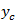is given by

Then the particular integral is

Where u and v are unknown and to be calculated using the formula

## Solved examples

Example-1: Solve the following DE by using variation of parameters-

Sol. We can write the given equation in symbolic form as-

To find CF-

It’s AE is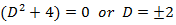So that CF is –To find PI-

Here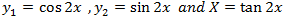Now

Thus PI will be

So that the complete solution is-

Example-2: Solve the following by using the method of variation of parameters.

Sol. This can be written as-

C.F.-

Auxiliary equation is-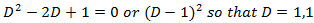So that the C.F. will be-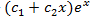P.I.-

Here-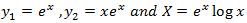Now

Thus

So that the complete solution is-

Interested in learning about similar topics? Here are a few hand-picked blogs for you!